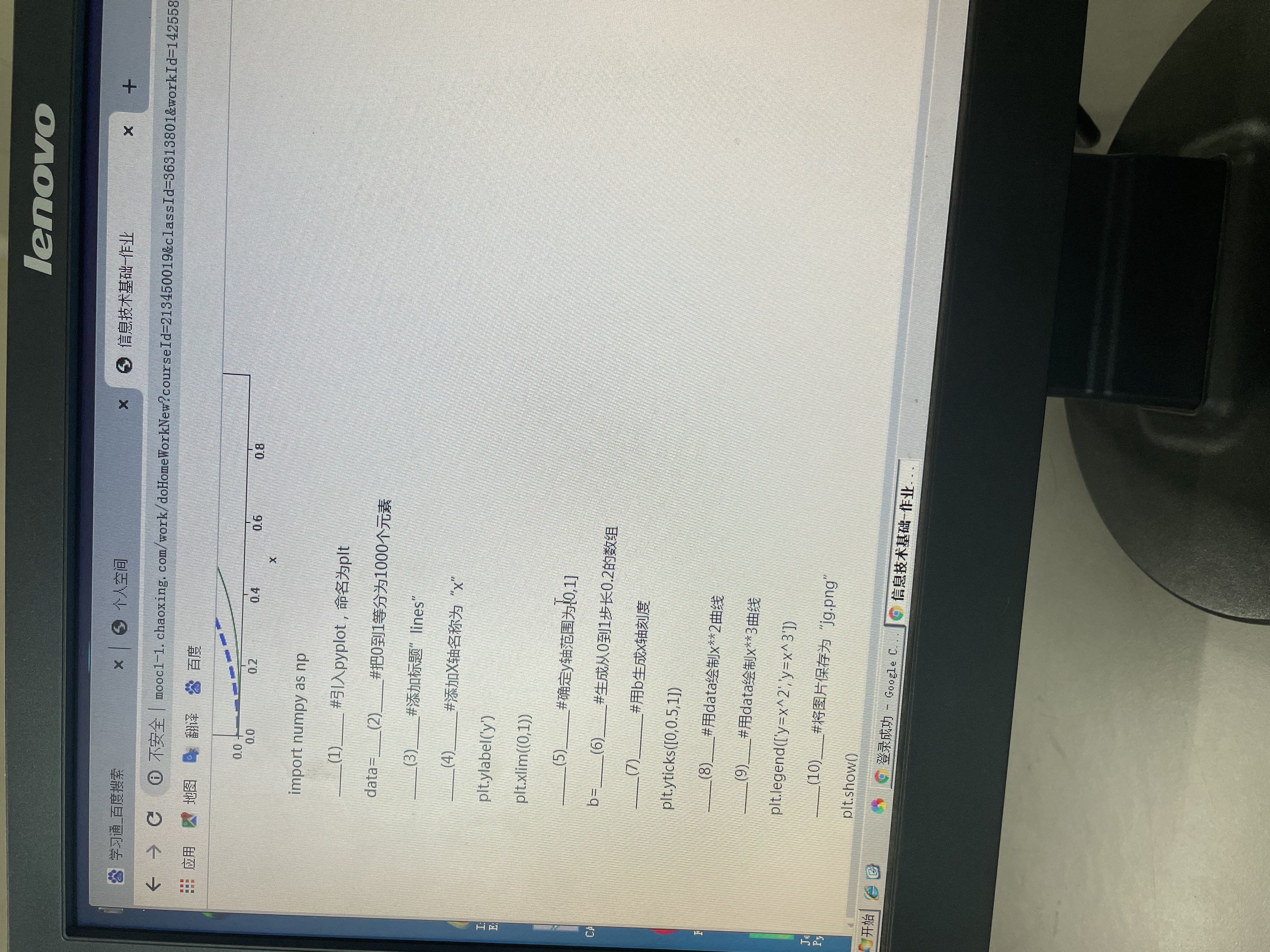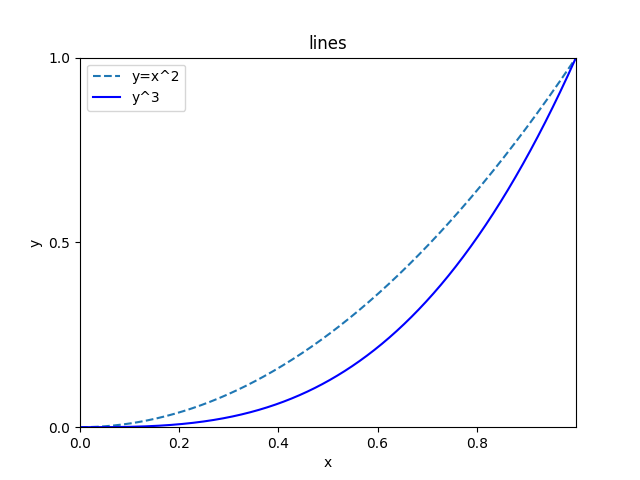2021-06-10 08:44

利用numpy和Matplotlib在同一张纸上绘制0-1间的x**2和x**3曲线• 写回答
• 好问题 提建议
• 追加酬金
• 关注问题
• 收藏
• 邀请回答

3条回答默认 最新

•盼小辉丶 2021-06-10 10:21
已采纳import numpy as np
import matplotlib.pyplot as plt

data = np.linspace(0,1,1000)
plt.title('lines')
plt.xlabel('x')
plt.ylabel('y')
plt.xlim((0,1))
plt.ylim((0,1))
b = np.arange(0,1,0.2)
plt.xticks(b)
plt.yticks([0,0.5,1])
plt.plot(data,data**2,ls='--')
plt.plot(data,data**3,color='b')
plt.legend(['y=x^2','y^3'])
plt.savefig('jp.png')
plt.show()
评论
解决 1 无用
打赏 举报